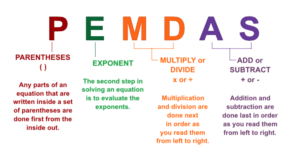# MS Math – Feb 7-18, 20226th Grade Math – Mrs. Evans

This week we are continue working in Chapter 7.  Last week we learned about exponents and how to write and evaluate numeric expressions using the proper order of operations.   This order can be summed up with the numonic:  PEMDAS, which is shown below:This week we will begin writing algebraic expression and learning how to evaluate those expressions for given values of the variables.  Then we will be learning to simplify expressions by combining like terms.  These skills will be the basis for algebraic studies.

Math Grade Math – Mrs. Evans and Mrs. VonFeldt

Last week we began our study of geometry in Module 8.  We learned about scale drawings and will use proportions to solve for dimensions.  Proportions are useful in many areas of math.  We last encountered them when we were evaluating rates.  Proportions can in a number of ways, the most direct being CROSS MULTIPLICATION.  Here is a video that discusses solving proportions:

This week we will be making observations about triangles.  We will learn about the Triangle Inequality Theorem which summarizes how we can tell if 3 lines will create a triangle.  We will also learn about the angles of a triangle.  We will be using a great program called GEOGEBRA, which is a great place for investigation.  Here is a link to the program:

https://www.geogebra.org/

8th Grade Math – Mrs. Ernest

The 8th graders will be practicing their algebra skills as we solve for missing angles in triangles, complimentary and supplementary angle problems. Students will be able to identify like terms (terms with X or without X) and move numbers from one side of an equation to the other. We will have a quiz on these skills later in the week.

Algebra – Mrs. VonFeldt

We have finished our study of systems of equations and systems of inequalities. Students should be able to evaluate a system and determine the most effective method to solve. There will be a test on Chapter 6 on Wednesday.© 2022 Assumption Catholic School | 2116 Cornwall Ave, Bellingham, WA 98225
Phone: 360.733.6133 | Fax: 360.647.4372
Email: theoffice@school.assumption.org
Dashboard | Web design and development by Olywebdev.com